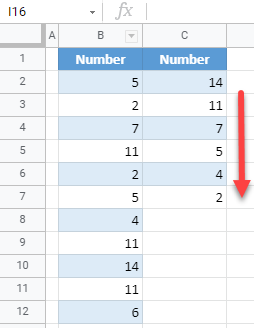# How to Sort Without Duplicates in Excel & Google Sheets

This tutorial demonstrates how to sort without duplicates in Excel and Google Sheets.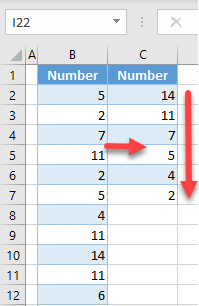## Sort Without Duplicates

Say you have the following list of numbers in Column B.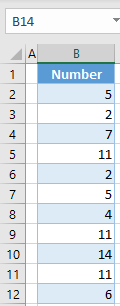As you can see, the numbers 2 and 5 are repeated twice, and the number 11 is repeated three times. To sort without including the duplicates, follow these steps:

1. First, identify the duplicate values in a helper column. In cell C2, enter the formula:
``=COUNTIF(B3:B\$12,"="&B2)``

The COUNTIF Function counts how many times a cell from range B3:B12 is equal to B2. This does a search for any other cell in range B3:B12, that has a value from B2 (5). In this case, the result is 1, since cell B7 is the value 5. Therefore, if a value from Column B doesn’t have duplicates, the result of the formula is 0.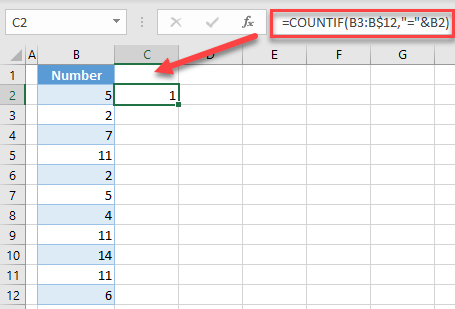1. Position the cursor in the bottom right corner of cell C2, until the cross appears.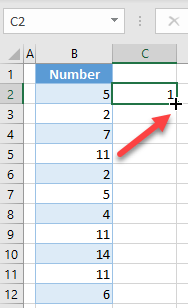1. Drag the formula down to the end of the range (C12).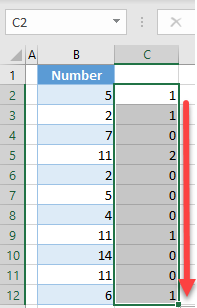1. The values with 0 in Column C, are those without duplicates. To include only these unique values, create another helper column; in cell D2, enter the formula:
``=IF(C2=0,B2,"")``

Use the IF Function to check if the value in C2 is 0 (doesn’t have duplicates). If true, copy the value from B2 to D2 and if not, leave D2 blank.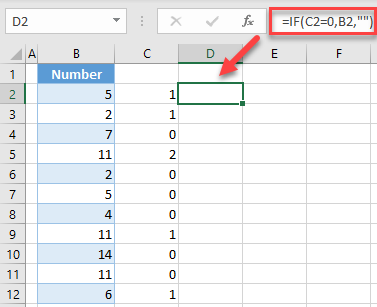1. Drag the formula down to cell D12. As you can see, Column D contains only unique values from Column B.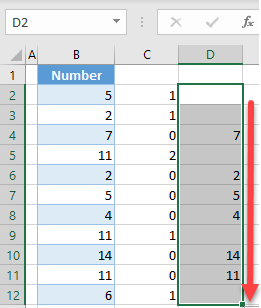1. Now create another helper column to sort values from Column D without blanks. In cell E2, enter the formula:
`=LARGE(\$D\$2:\$D\$12,ROW(D2)-ROW(D\$2)+1)`

Use the LARGE Function to determine a position of a value in an array. In this case, the array is \$D\$2:\$D\$12. For the position in the array, use the ROW Function:

``ROW(D2) - ROW(D\$2) + 1``

This piece of the formula increases by one for every new row. For example, cell E2 returns position 1 from array D2:D12 using the LARGE Function, which is D2 (14); E3 returns position 2 (D3), etc.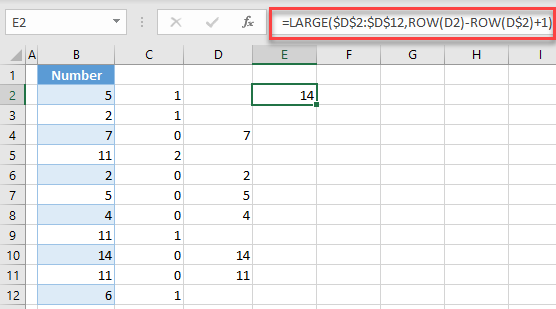1. Drag the formula down to cell E12.
Now all values from Column B are sorted in descending order in Column E. If you want to sort from smallest to largest, you can use the same formula, just with the SMALL Function instead:
``=SMALL(\$D\$2:\$D\$12,ROW(D2)-ROW(D\$2)+1)``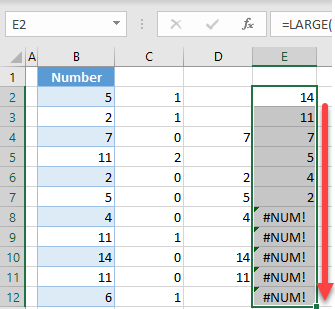1. Finally, you can copy and paste as values in Column E, delete helper Columns C and D, and delete error values (#NUM!) caused by empty cells.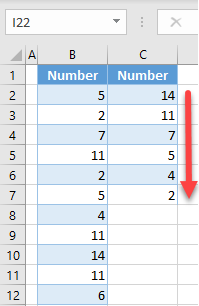## Sort Without Duplicates in Google Sheets

You can use the exact same steps used in Excel to sort without duplicates in Google Sheets. The final output is the same as in Excel.